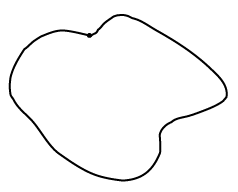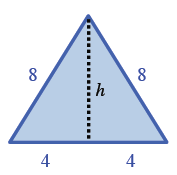### Home > CCG > Chapter 8 > Lesson 8.3.3 > Problem8-126

8-126.Christie has tied a string that is $24$ cm long into a closed loop, like the one at right.

1. She decided to form an equilateral triangle with her string. What is the area of the triangle?

The length of each side will be $8$ cm. Use the Pythagorean Theorem to solve for the height of the triangle, which will then allow you to calculate its area.2. She then forms a square with the same loop of string. What is the area of the square? Is it more or less than the equilateral triangle she created in part (a)?

The length of each side of the square will be $6$ cm.

3. If she forms a regular hexagon with her string, what would be its area? Compare this area with the areas of the square and equilateral triangle from parts (a) and (b).

Each side of the hexagon will be $4$ cm. A hexagon is made of six equilateral triangles, so use the hint in part (a) to calculate the area of one equilateral triangle and then multiply it by $6$.

4. What shape should Christie form to enclose the greatest area?

The more sides a shape has, the greater its area.

A circle will have the greatest area.